Advanced search [ New messages · Forum rules · Members ]
 Forum » SpaceEngine » Mods and Addons » Changing the shaders parameters
 Rodrigo Date: Saturday, 17.03.2012, 03:17 | Message # 1 Explorer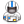Group: Local Moderators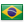Brazil Messages: 285 Status: Offline I was trying to make more realistic canyons, so I changed the shaders files, and I got this: before:after:I think it looks so much better now, because there are long rifts and valleys, just like on mars. I´d love to have some feedback on this, especially from Spaceengineer. Please look at the code and see if I made something stupid. this is tg_terra_height.glsl Code #ifdef _VERTEX_ void main() {      gl_Position = ftransform();      gl_TexCoord = gl_MultiTexCoord0; } #else float   HeightMapTerra(vec3 point) {      noiseH          = 0.5;      noiseLacunarity = 2.218281828459;      noiseOffset     = 0.8;      float massif, global;      vec3  distort;      vec3  p = point * mainFreq + Randomize;      // Global landscape      noiseOctaves = 5;      distort = 0.35 * fBmVec3(p * 2.37);      noiseOctaves = 4;      distort += 0.005 * (1.0 - abs(fBmVec3(p * 132.3)));      global = 1.0 - Cell3Noise(p + distort);      // Venus-like structure      float venus = 0.0;      if (venusMagn > 0.05)      {          noiseOctaves = 4;          distort = fBmVec3(point * 0.3) * 1.5;          noiseOctaves = 6;          venus = fBm((point + distort) * venusFreq) * venusMagn;      }      global += venus;      global = (global - seaLevel) * 0.5 + seaLevel;      // Dunes      float dunes = 0.0;      if (dunesSqrtDensity > 0.05)      {          noiseOctaves = 3;          massif = Cell2Noise(point * 3.5 + 0.2 * fBmVec3(point*5.12));          massif = 1.0 - smoothstep(0.0, 1.0, massif / dunesSqrtDensity);          if (massif > 0.0)          {              noiseOctaves = 5.0;              dunes = dunesFreq + fBm(p * 3.21);              dunes = max(fBm(p * dunes) + 0.7, 0.0);              dunes = dunesMagn * massif * dunes;          }      }      // "Eroded" hills      float hills = 0.0;      if (hillsSqrtDensity > 0.05)      {          noiseOctaves = 3;          massif = Cell2Noise(point * 4.0 + 0.2 * fBmVec3(point*5.12));          massif = 1.0 - smoothstep(0.0, 1.0, massif / hillsSqrtDensity);          if (massif > 0.0)          {              noiseOctaves = 10.0;              noiseH       = 1.0;              noiseOffset  = 1.0;              hills = hillsMagn * massif * (1.5 - RidgedMultifractal(point * hillsFreq + Randomize, 2.0));              //noiseOctaves = 10.0;              //distort = 0.2 * fBmVec3(p * 15.2);              //vec2 cell = Cell3Noise2(25.51 * (p + distort));              //hills = smoothstep(0.0, 1.0, abs(cell.y - cell.x));          }      }      // Canyons      float canyon = 0.0;      if (canyonSqrtDensity > 0.05)      {          noiseOctaves = 3;          massif = Cell2Noise(point * 3.8 + 0.2 * fBmVec3(point*5.12));          massif = 1.0 - smoothstep(0.0, 1.0, massif / canyonSqrtDensity);          if (massif > 0.0)          {              noiseOctaves = 10;              canyon = 1.5 * (hillsMagn * massif * 3 * (1.5 - RidgedMultifractal(point * hillsFreq + Randomize, 2.0))) + (montesMagn * massif * 5 * RidgedMultifractal(point * montesFreq + Randomize, 2.0));                noiseH       = 1.0;              noiseOffset  = 1.0;                //canyonFreq + 30.0 * fBm(p * 1.321);              float canyonWidth = clamp(1.0 - 1.5 * massif, 0.3, 2.0);              canyon = pow(abs(fBm(p * 3 * canyon)), canyonWidth);              canyon = smoothstep(0.0, 1.0, (canyon - 0.5) * 6) - 1.0;              canyon = canyon * massif * canyonMagn;          }      }      global += canyon + (dunes + hills) * clamp(70 * (global - seaLevel), 0.0, 1.0);      // Mare      float mare = global;      if (mareSqrtDensity > 0.05)      {          craterDistortion = 1.0;          noiseOctaves = 6;  // Mare roundness distortion          mare = MareNoise(point, global, 0.0);      }      // Craters      float crater = 0.0;      if (craterSqrtDensity > 0.05)      {          noiseOctaves = 3;  // Craters roundness distortion          craterDistortion = 1.0;          craterRoundDist  = 0.03;          heightFloor = -0.1;          heightPeak  = 0.6;          heightRim   = 1.0;          crater = CraterNoise(point, 0.5 * craterMagn, craterFreq, craterSqrtDensity, craterOctaves);          noiseOctaves = 10.0;          noiseH       = 1.0;          noiseOffset  = 1.0;          crater = RidgedMultifractalDetail(point * 0.3 * montesFreq + Randomize, 2.0, 0.25 * crater);      }      // Mountains      float montes = 0.0;      if (montesSqrtDensity > 0.05)      {          noiseOctaves = 3;          massif = Cell2Noise(point * 4.5 + 0.2 * fBmVec3(point*5.12));          massif = 1.0 - smoothstep(0.0, 1.0, massif / montesSqrtDensity);          //massif = saturate((global - seaLevel - 0.05) / 0.07) * 2.0 - 1.0;          //massif = 1.0 - montes*montes;          if (massif > 0.0)          {              noiseOctaves = 10.0;              noiseH       = 1.0;              noiseOffset  = 1.0;              montes = montesMagn * massif * RidgedMultifractal(point * montesFreq + Randomize, 2.0);          }      }      //float height = mare + crater + montes;      float height = mare + crater + montes * clamp(5 * (global - seaLevel), 0.0, 1.0);      // Assign a climate type      noiseOctaves = (cloudsStyle == 1.0) ? 5.0 : 12.0;      noiseH          = 0.5;      noiseLacunarity = 2.218281828459;      noiseOffset     = 0.8;      float climate, latitude;      if (tidalLock == 0.0)      {          latitude = abs(point.y);          latitude += 0.15 * (fBm(point * 0.7 + Randomize) - 1.0);          latitude = saturate(latitude);      }      else      {          latitude = 1.0 - point.x;          latitude += 0.15 * (fBm(point * 0.7 + Randomize) - 1.0);      }      // Ice caps      float edge;      if (cloudsStyle == 1.0)      {          edge = saturate(15.0 * (latitude - latIceCaps));          height = height * 0.1 + icecapHeight * edge;      }      else      {          edge = saturate(3.0 * (latitude - latIceCaps));          height += icecapHeight * sqrt(edge);      }      return height; } void main() {      vec3  point = GetSurfacePoint();      //float height = max((HeightMapTerra(point) - seaLevel) / (1.0 + montesMagn), 0.0);      float height = HeightMapTerra(point);      gl_FragColor = vec4(height); } #endif
 Rodrigo Date: Saturday, 17.03.2012, 03:28 | Message # 2 ExplorerGroup: Local ModeratorsBrazil Messages: 285 Status: Offline hmm.... I was flying around some places, and I guess thre´s too much noise. I´ll try to soften it.
 Rodrigo Date: Saturday, 17.03.2012, 03:52 | Message # 3 ExplorerGroup: Local ModeratorsBrazil Messages: 285 Status: Offline I dunno, maybe this is a little better:Code #ifdef _VERTEX_ void main() {         gl_Position = ftransform();         gl_TexCoord = gl_MultiTexCoord0; } #else float   HeightMapTerra(vec3 point) {         noiseH          = 0.5;         noiseLacunarity = 2.218281828459;         noiseOffset     = 0.8;         float massif, global;         vec3  distort;         vec3  p = point * mainFreq + Randomize;         // Global landscape         noiseOctaves = 5;         distort = 0.35 * fBmVec3(p * 2.37);         noiseOctaves = 4;         distort += 0.005 * (1.0 - abs(fBmVec3(p * 132.3)));         global = 1.0 - Cell3Noise(p + distort);         // Venus-like structure         float venus = 0.0;         if (venusMagn > 0.05)         {             noiseOctaves = 4;             distort = fBmVec3(point * 0.3) * 1.5;             noiseOctaves = 6;             venus = fBm((point + distort) * venusFreq) * venusMagn;         }         global += venus;         global = (global - seaLevel) * 0.5 + seaLevel;         // Dunes         float dunes = 0.0;         if (dunesSqrtDensity > 0.05)         {             noiseOctaves = 3;             massif = Cell2Noise(point * 3.5 + 0.2 * fBmVec3(point*5.12));             massif = 1.0 - smoothstep(0.0, 1.0, massif / dunesSqrtDensity);             if (massif > 0.0)             {                 noiseOctaves = 5.0;                 dunes = dunesFreq + fBm(p * 3.21);                 dunes = max(fBm(p * dunes) + 0.7, 0.0);                 dunes = dunesMagn * massif * dunes;             }         }         // "Eroded" hills         float hills = 0.0;         if (hillsSqrtDensity > 0.05)         {             noiseOctaves = 3;             massif = Cell2Noise(point * 4.0 + 0.2 * fBmVec3(point*5.12));             massif = 1.0 - smoothstep(0.0, 1.0, massif / hillsSqrtDensity);             if (massif > 0.0)             {                 noiseOctaves = 10.0;                 noiseH       = 1.0;                 noiseOffset  = 1.0;                 hills = hillsMagn * massif * (1.5 - RidgedMultifractal(point * hillsFreq + Randomize, 2.0));                 //noiseOctaves = 10.0;                 //distort = 0.2 * fBmVec3(p * 15.2);                 //vec2 cell = Cell3Noise2(25.51 * (p + distort));                 //hills = smoothstep(0.0, 1.0, abs(cell.y - cell.x));             }         }         // Canyons         float canyon = 0.0;         if (canyonSqrtDensity > 0.05)         {             noiseOctaves = 3;             massif = Cell2Noise(point * 3.8 + 0.2 * fBmVec3(point*5.12));             massif = 1.0 - smoothstep(0.0, 1.0, massif / canyonSqrtDensity);             if (massif > 0.0)             {                 noiseOctaves = 10;                 canyon = 1.8 * (hillsMagn * massif * 3 * (1.5 - RidgedMultifractal(point * hillsFreq + Randomize, 2.0))) + (montesMagn * massif * 5 * RidgedMultifractal(point * montesFreq + Randomize, 2.0));                      noiseH       = 1.5;                 noiseOffset  = 1.0;                      //canyonFreq + 30.0 * fBm(p * 1.321);                 float canyonWidth = clamp(1.0 - 1.5 * massif, 0.3, 2.0);                 canyon = pow(abs(fBm(p * 3 * canyon)), canyonWidth);                 canyon = smoothstep(0.0, 1.0, (canyon - 0.5) * 6) - 1.0;                 canyon = canyon * massif * canyonMagn;             }         }         global += canyon + (dunes + hills) * clamp(70 * (global - seaLevel), 0.0, 1.0);         // Mare         float mare = global;         if (mareSqrtDensity > 0.05)         {             craterDistortion = 1.0;             noiseOctaves = 6;  // Mare roundness distortion             mare = MareNoise(point, global, 0.0);         }         // Craters         float crater = 0.0;         if (craterSqrtDensity > 0.05)         {             noiseOctaves = 3;  // Craters roundness distortion             craterDistortion = 1.0;             craterRoundDist  = 0.03;             heightFloor = -0.1;             heightPeak  = 0.6;             heightRim   = 1.0;             crater = CraterNoise(point, 0.5 * craterMagn, craterFreq, craterSqrtDensity, craterOctaves);             noiseOctaves = 10.0;             noiseH       = 1.0;             noiseOffset  = 1.0;             crater = RidgedMultifractalDetail(point * 0.3 * montesFreq + Randomize, 2.0, 0.25 * crater);         }         // Mountains         float montes = 0.0;         if (montesSqrtDensity > 0.05)         {             noiseOctaves = 3;             massif = Cell2Noise(point * 4.5 + 0.2 * fBmVec3(point*5.12));             massif = 1.0 - smoothstep(0.0, 1.0, massif / montesSqrtDensity);             //massif = saturate((global - seaLevel - 0.05) / 0.07) * 2.0 - 1.0;             //massif = 1.0 - montes*montes;             if (massif > 0.0)             {                 noiseOctaves = 10.0;                 noiseH       = 1.0;                 noiseOffset  = 1.0;                 montes = montesMagn * massif * RidgedMultifractal(point * montesFreq + Randomize, 2.0);             }         }         //float height = mare + crater + montes;         float height = mare + crater + montes * clamp(5 * (global - seaLevel), 0.0, 1.0);         // Assign a climate type         noiseOctaves = (cloudsStyle == 1.0) ? 5.0 : 12.0;         noiseH          = 0.5;         noiseLacunarity = 2.218281828459;         noiseOffset     = 0.8;         float climate, latitude;         if (tidalLock == 0.0)         {             latitude = abs(point.y);             latitude += 0.15 * (fBm(point * 0.7 + Randomize) - 1.0);             latitude = saturate(latitude);         }         else         {             latitude = 1.0 - point.x;             latitude += 0.15 * (fBm(point * 0.7 + Randomize) - 1.0);         }         // Ice caps         float edge;         if (cloudsStyle == 1.0)         {             edge = saturate(15.0 * (latitude - latIceCaps));             height = height * 0.1 + icecapHeight * edge;         }         else         {             edge = saturate(3.0 * (latitude - latIceCaps));             height += icecapHeight * sqrt(edge);         }         return height; } void main() {         vec3  point = GetSurfacePoint();         //float height = max((HeightMapTerra(point) - seaLevel) / (1.0 + montesMagn), 0.0);         float height = HeightMapTerra(point);         gl_FragColor = vec4(height); } #endif Edited by Rodrigo - Saturday, 17.03.2012, 06:06
 mustafa2812 Date: Saturday, 17.03.2012, 04:33 | Message # 4Space Pilot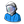Group: Users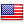United States Messages: 121 Status: Offline Those look great! PC specs: Windows 7 64 bit. Intel Core i5-2400 quad-core (3.1GHz, 6MB Cache). 6GB DDR3-1333MGz SDRAM[2 DIMMs]. 1 TB 7200 rpm SATA 3Gb/s hard drive. 3GB DDR3 NVIDIA GeForce GT 545.
 Rodrigo Date: Saturday, 17.03.2012, 05:29 | Message # 5 ExplorerGroup: Local ModeratorsBrazil Messages: 285 Status: Offline Thanks! Edited by Rodrigo - Saturday, 17.03.2012, 05:59
 Rodrigo Date: Saturday, 17.03.2012, 19:59 | Message # 6 ExplorerGroup: Local ModeratorsBrazil Messages: 285 Status: Offline Did anyone else try this?
 Duke Date: Saturday, 17.03.2012, 21:37 | Message # 7 Space Pilot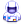Group: SE team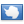Antarctica Messages: 88 Status: Offline I've tried. looks good. Edited by Duke - Saturday, 17.03.2012, 21:42
 Freak2121 Date: Sunday, 18.03.2012, 03:16 | Message # 8Space PilotGroup: Users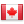Canada Messages: 89 Status: Offline These are pretty good.I messed with clouds a bit, right now I'm using this: Code#ifdef _VERTEX_ void main() {      gl_Position = ftransform();      gl_TexCoord = gl_MultiTexCoord0; } #else float   HeightMapClouds(vec3 point) {      //return 0.0;      float zone = cos(point.y);      float ang = zone * twistMagn;      float weight = 1.0;      float offset = 0.0;      vec3  twistedPoint = point;      // Compute cyclons      vec3  cycloneCenter;      float cycloneRadius = 1.0;      float cycloneAmpl;      if (tidalLock != 0.0)      {          cycloneCenter = vec3(0.0, 1.0, 0.0);          cycloneRadius = length(cycloneCenter - point) / cycloneSqrtDensity;          cycloneAmpl   = -cycloneMagn * sign(cycloneCenter.y);      }      else      {          vec4 cell = Cell2NoiseVec(point * cycloneFreq);          cycloneCenter = cell.xyz;          cycloneRadius = length(cycloneCenter - point) / cycloneSqrtDensity;          cycloneAmpl   = 0.8*pi * sign(cycloneCenter.y);      }      if (cycloneRadius < 1.0)      {          float dist = 1.0 - cycloneRadius;          float fi = mix(log(cycloneRadius), pow(dist, 3.0), cycloneRadius);          twistedPoint = Rotate(cycloneAmpl*fi, cycloneCenter, point);          if (cycloneRadius < 0.05)              weight = 1.0 - pow(1.0 - cycloneRadius / 0.05, 2);          else              weight = 1.0;          weight = weight * (1.0 + dist);          //offset += 0.7 * dist;      }      // Compute Coriolis effect      float sina = sin(ang);      float cosa = cos(ang);      twistedPoint = vec3(cosa*twistedPoint.x-sina*twistedPoint.z, twistedPoint.y, sina*twistedPoint.x+cosa*twistedPoint.z);      twistedPoint = twistedPoint * cloudsFreq + Randomize;      // Compute flow-like distortion      noiseH          = 0.75;      noiseLacunarity = 5.5;      noiseOffset     = 0.8;      noiseOctaves = 4;      vec3  distort = fBmVec3(twistedPoint * 0.75) * 1.5;      noiseOctaves = 6;      float global = (fBm(twistedPoint + distort) + 1.0) * 0.7;      global = (global + offset) * weight;      // Compute turbilence features      noiseOctaves = cloudsOctaves;      float turbulence = fBm(point * 100.0 * cloudsFreq + Randomize) * 0.1;      return  global + turbulence * step(0.1, global); } void main() {      vec3  point = GetSurfacePoint();      float height = HeightMapClouds(point);      gl_FragColor = vec4(height); } #endif Don't know if it's any better or not, but I definitely think we should work together to improve clouds. They're a bit lacking right now. Intel Core i5 @ 4.534GHz 8GBs of DDR3 RAM @ 1600mhz EVGA GTX970 SSC Windows 7 64-bit
 SpaceEngineer Date: Sunday, 18.03.2012, 14:14 | Message # 9Author of Space Engine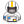Group: Administrators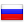Russian Federation Messages: 4800 Status: Offline Please mark your changes in commets, I don't want to simply replace my shaders, but to look at your changes. *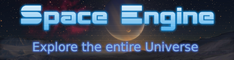Rodrigo Date: Monday, 19.03.2012, 12:12 | Message # 10 ExplorerGroup: Local ModeratorsBrazil Messages: 285 Status: Offline Ok I changed this line of code on //canyons "       >>> noiseOctaves = 10;       >>>   canyon = 1.8 * (hillsMagn * massif * 3 * (1.5 - RidgedMultifractal(point * hillsFreq + Randomize, 2.0))) + (montesMagn * massif * 5 * RidgedMultifractal(point * montesFreq + Randomize, 2.0));             >>> noiseH       = 1.5;       >>>   noiseOffset  = 1.0;                      //canyonFreq + 30.0 * fBm(p * 1.321);                 float canyonWidth = clamp(1.0 - 1.5 * massif, 0.3, 2.0);      >>>    canyon = pow(abs(fBm(p * 3 * canyon)), canyonWidth);                 canyon = smoothstep(0.0, 1.0, (canyon - 0.5) * 6) - 1.0;                 canyon = canyon * massif * canyonMagn;             }         } " As gou see, i mixed some parameters from mountains & eroded hills, and made some random changes until it looked good! It looks now that there's some erosion effect from water or any liquid. Ps: i am no programmer, that's why I asked to you to see if I made something stupid with your code.Rodrigo Date: Tuesday, 20.03.2012, 02:36 | Message # 11 ExplorerGroup: Local ModeratorsBrazil Messages: 285 Status: Offline Double post Edited by Rodrigo - Tuesday, 20.03.2012, 02:55
 SpaceEngineer Date: Wednesday, 21.03.2012, 11:43 | Message # 12Author of Space EngineGroup: AdministratorsRussian Federation Messages: 4800 Status: Offline Hmmm, I can implement supporting of custom shaders for user-made planets. I.e. you can make a planetary system, assigning your textures for planets, and to write your own shaders for it. But these shaders will work only on your planets, the rest of the Universe will be unchanged. *HarbingerDawn Date: Wednesday, 21.03.2012, 19:15 | Message # 13Cosmic CuratorGroup: AdministratorsUnited States Messages: 8717 Status: Offline Quote (SpaceEngineer)Hmmm, I can implement supporting of custom shaders for user-made planets. I.e. you can make a planetary system, assigning your textures for planets, and to write your own shaders for it. But these shaders will work only on your planets, the rest of the Universe will be unchanged. This is a great idea. It would give people the freedom to experiment, express their ideas and make their own visions come to life, while maintaining a consistent procedural universe for all users. All forum users, please read this! My SE mods and addons Phenom II X6 1090T 3.2 GHz, 16 GB DDR3 RAM, GTX 970 3584 MB VRAM
 Forum » SpaceEngine » Mods and Addons » Changing the shaders parameters
• Page 1 of 1
• 1
 Search: